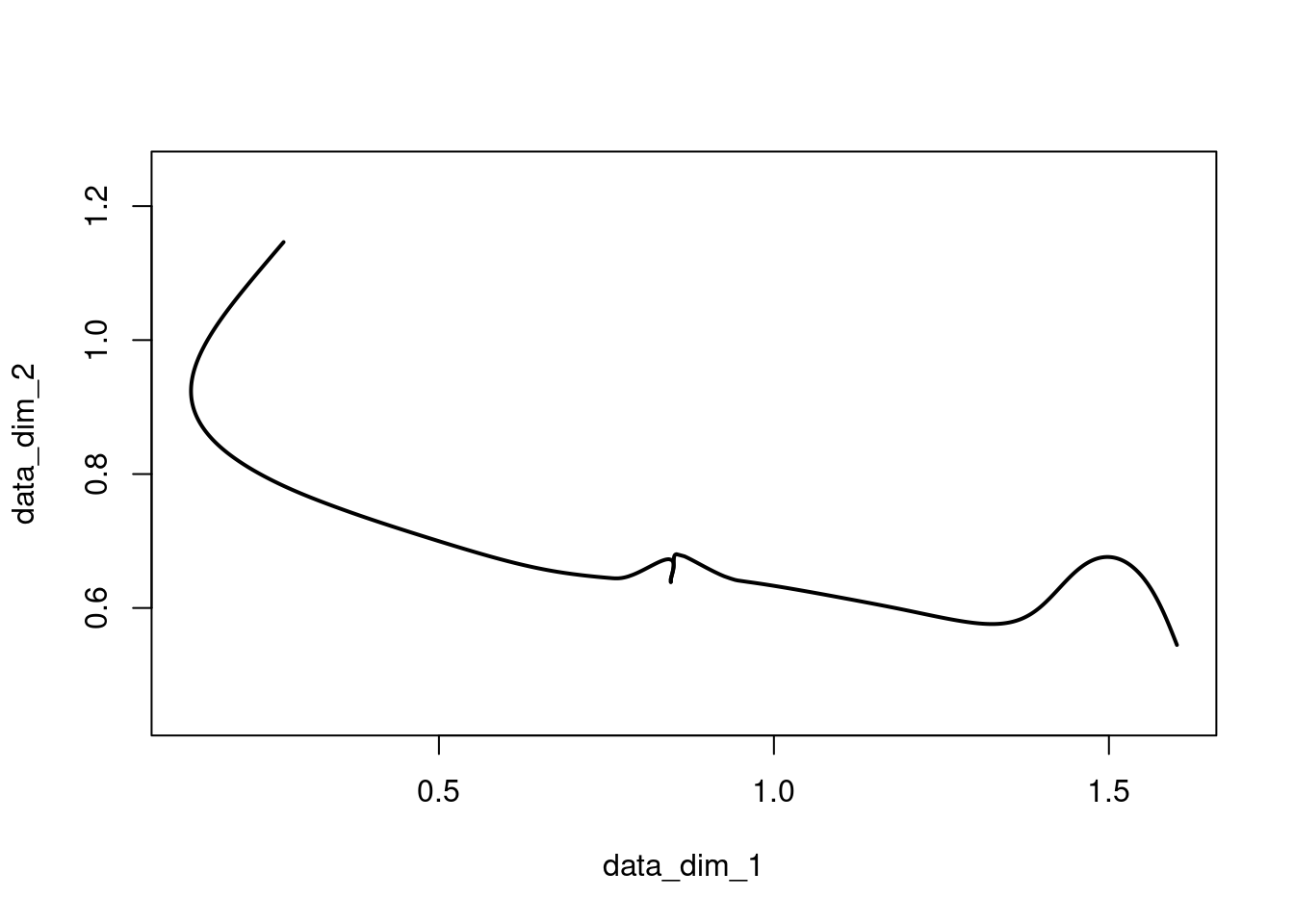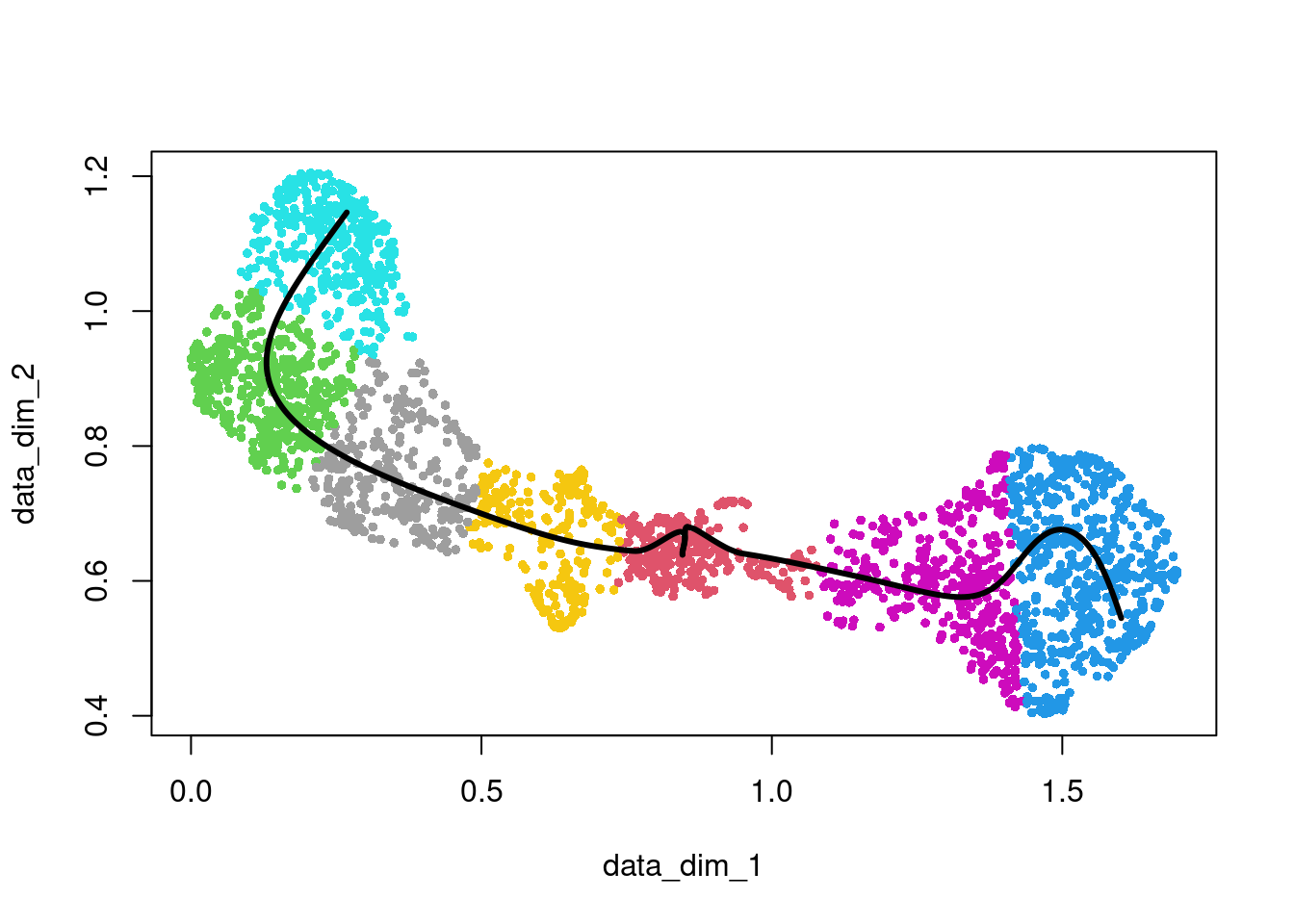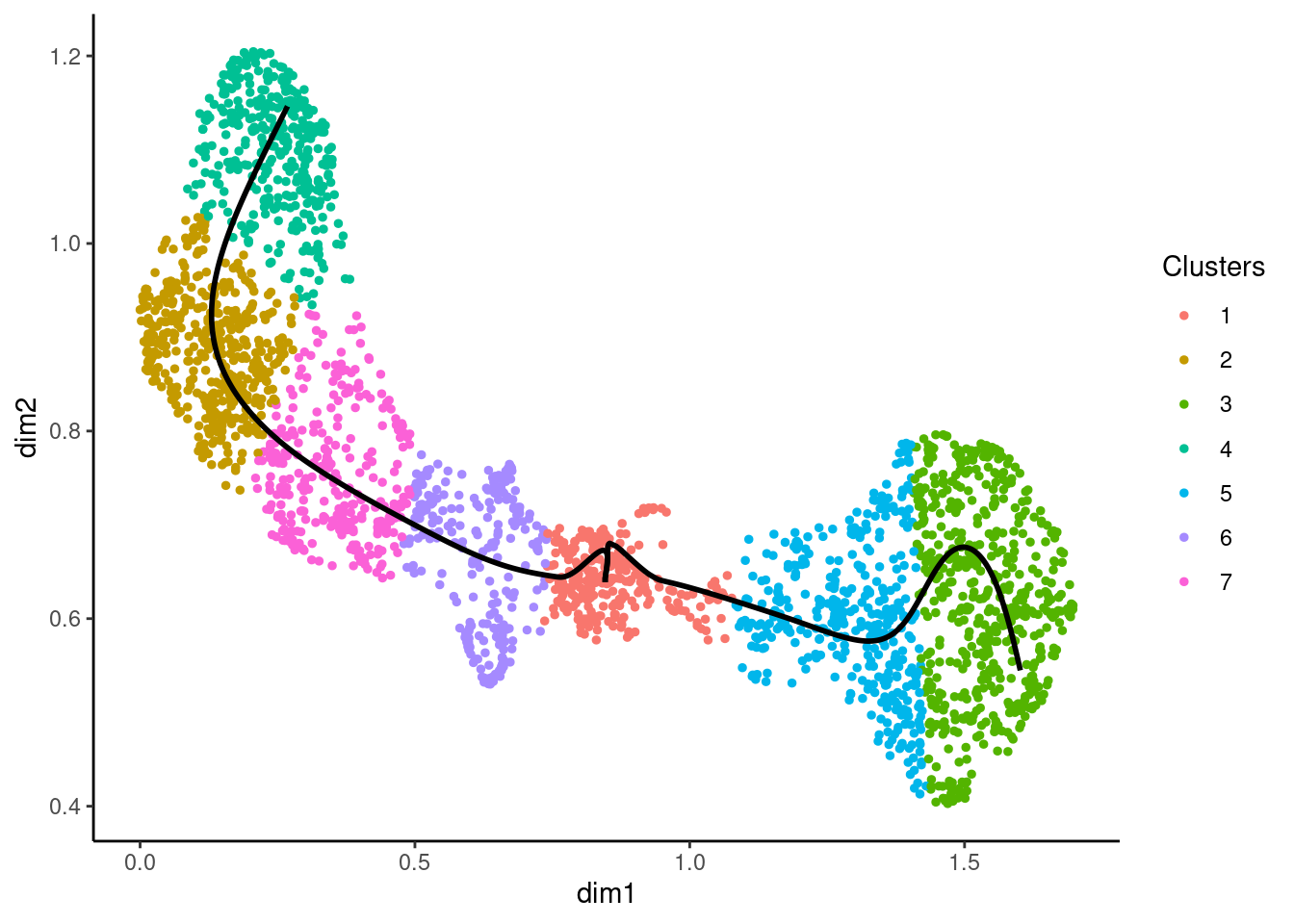# Introduction

The `traviz` package was created to collect visualization functions related to trajectory inference. Asides from general purpose functions useful to any user, it also contains visualizations that can be used in a `slingshot`, `tradeSeq` or `condiments` workflow.

# Visualization of a trajectory

First, we will demonstrate functions to visualize a trajectory. Here, we’ll work with a trajectory as estimated by Slingshot (Street et al. 2018). An example trajectory is provided along with the `traviz` package.

Below, we show how one can use the `lines` and `plot` functions for a `SlingshotDataSet` object. We also show how the `plotGeneCount` function can be used for a quick visualization of the trajectory.

``````library(slingshot)
library(traviz)``````
``````##
## Attaching package: 'traviz'``````
``````## The following object is masked from 'package:scater':
##
##     plotExpression``````
``````data(crv, package = "traviz")
class(crv)``````
``````##  "SlingshotDataSet"
## attr(,"package")
##  "slingshot"``````
``````rd <- slingReducedDim(crv)
cl <- apply(slingClusterLabels(crv),1, function(x) which(x==1))

## Only visualize the trajectory
plot(crv)````````````## Visualize the trajectory on top of cells in reduced space
plot(rd, pch=16, col=cl+1, cex=2/3)
lines(crv, col="black", lwd=3)````````````## Visualizing trajectory and clusters using plotGeneCount
plotGeneCount(crv, clusters=cl)``````### 3D visualization

The same functions (i.e., `plot` and `lines`) can also be used to visualize trajectories in 3D space using the `rgl` package. This can be done using the `plot3d` and `lines3d` functions, in similar vein as the 2D visualizations above.

# Visualization of gene expression along a trajectory

## Visualizing gene expression along with the trajectory in reduced space

The `plotGeneCount` also allows you to visualize the trajectory in reduced space, where each cell is colored according to its expression of the gene as defined by the `gene` argument.

``````data(counts, package="traviz")
plotGeneCount(crv, counts, gene = "Mpo")``````